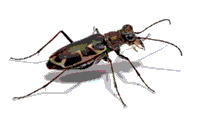## Entomology, Department of## Faculty Publications: Department of Entomology

#### Date of this Version

2003

Published in J. Econ. Entomol. 96(3): 536-542 (2003) Copyright 2003 by the Entomological Society of America Used by Permission

#### Abstract

Despite the recognition that the economic injury level (EIL) is determined by dynamic biological and economic parameters, which can be highly variable and uncertain, there has been little effort to quantify uncertainty and to use estimates of uncertainty in the determination of EILs. In this paper, we define the probabilistic EIL (PEIL) and develop PEILs for two insect pest scenarios: alfalfa weevil larvae, Hypera postica (Gyllenhal), on early bud-stage alfalfa, and bean leaf beetle adults, Cerotoma trifurcata (Forster), on V1-stage soybean. The PEIL is an EIL that reflects its probability of occurrence. The probability of occurrence is determined by incorporating the uncertainty associated with the input variables used to calculate the EIL. We used Monte Carlo simulation, a random sampling technique in which each input variable in the model was sampled repeatedly from a range of possible values based on probability distributions. Each input variable's probability distribution was sampled such that the distribution's shape was reproduced. Then, the variability for each input was propagated into the output of the model so that the model output reflected the probability of values that could occur. This represents the first use of the Monte Carlo technique to determine EILs.

COinS